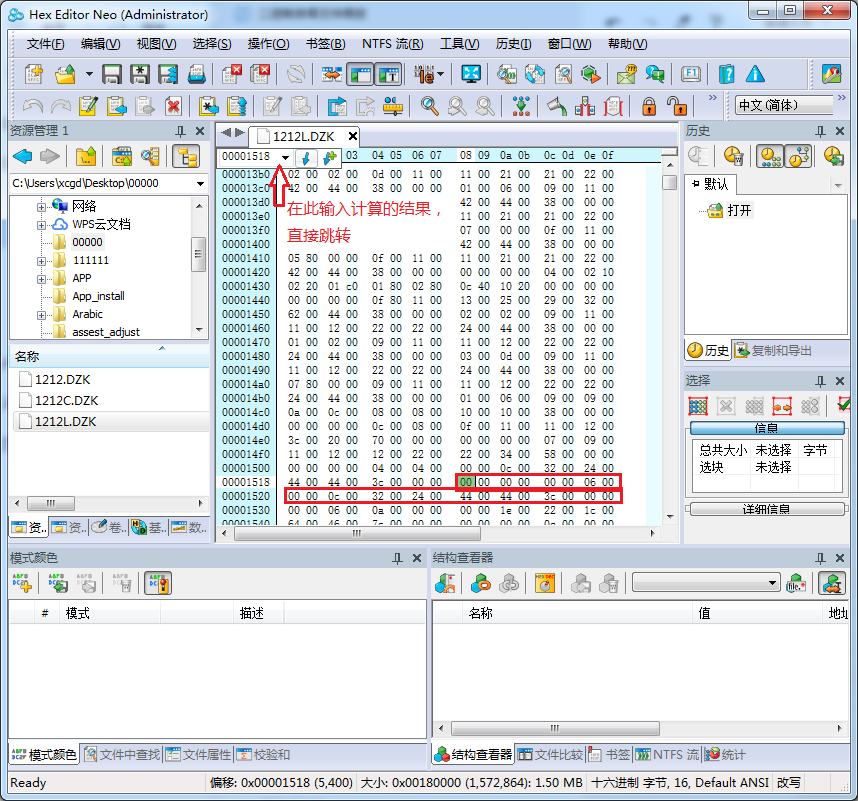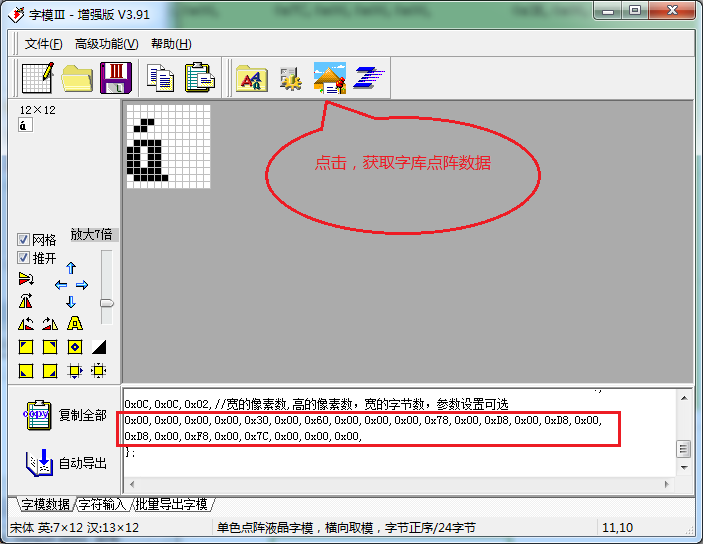# Modification scheme of binary files in dot matrix font library

Preface: This article aims at the complete Unicode dot matrix font library (other font library calculation methods are different)
solution: part of the dot matrix font library does not meet the business requirements and needs to be changed again (not the whole font library)

Steps:

1. Get the Unicode code of the character to be modified
(example: á Unicode code 0x00e1)

2. Calculate the position of characters in binary file;

Calculation formula: index = number of bytes occupied by Unicode * characters (need to be converted to decimal and then converted to hexadecimal)

In this example, (0x00e1) converts 225225 * 24 (bytes occupied by characters in the font) = 5400 to hexadecimal

1518）

3. According to the calculated position, find the corresponding data (24 consecutive bits after the position (12 * 2)):

As shown in the picture

The red box is the data of this character in the font,4. Get the desired character lattice data5. Replace the original data, complete the

Software resources: